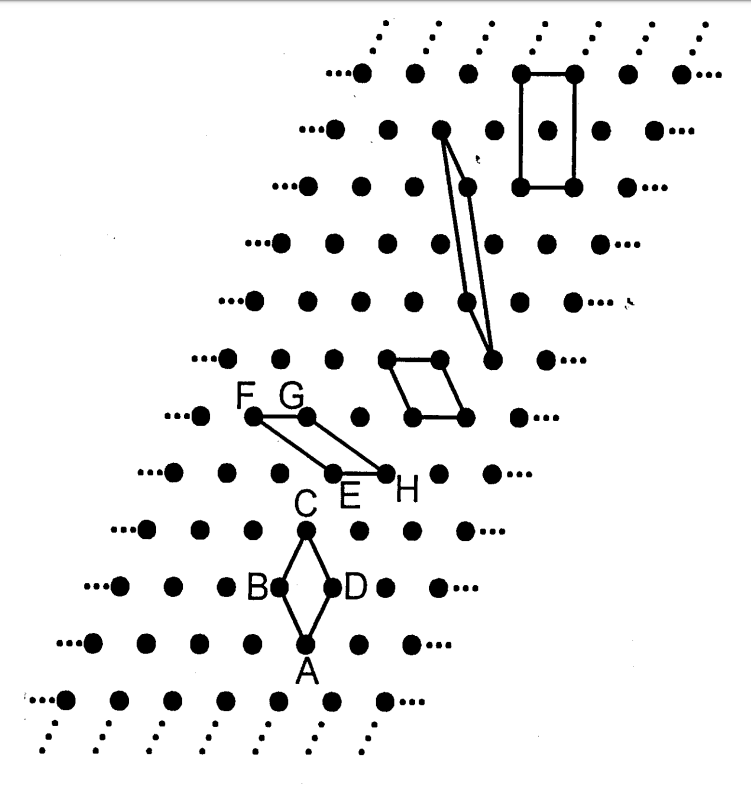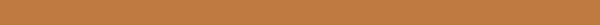# Niggli reduce cell of 2D and 3D lattice

## Code

https://github.com/unkcpz/pniggli

## Introduction

Correct identification of the Bravais lattice of a crystal is an important early step in structure solution. Niggli reduction is a commonly used technique.

Examples in two dimensional lattice.An infinite variety of unit cells, vastly different in appearance and specification, can generate the same mathematical lattice.

## Algorithm to determin Niggli cell(2D)

The following variables used in this algorithm are initialized for step A1-A4.

Define following variables as:

$$A=\bf{a\cdot a}$$ $$B=\bf{b\cdot b}$$ $$Y = \gamma = 2\bf{a\cdot b}$$

They are elements of metric tensor of $G$: $$G=\begin{pmatrix} A & \gamma/2 \\ \gamma/2 & B \\ \end{pmatrix}$$

$M$ represent the transformation matrix that is applied to basis vectors. The lattice vectors is represented as row vectors: $$L= \begin{pmatrix} \bf{a} \\ \bf{b} \end{pmatrix} = \begin{pmatrix} a_x & a_y \\ b_x & b_y \end{pmatrix}$$

$M$ operated as left transformation matrix to lattice vector $L$: $$\mathrm{new}\quad L = M \cdot L$$ that is, $$\begin{pmatrix} \bf{a'} \\ \bf{b'} \end{pmatrix} = M_{2\times 2} \cdot \begin{pmatrix} \bf{a} \\ \bf{b} \end{pmatrix}$$

### Step 1

Ensure that basis length, $|\bf{a}| < |\bf{b}|$ that is $A < B$

If $A>B+eps$, let $(\bf{a'}, \bf{b'}) = (\bf{b}, \bf{a})$

$$M = \begin{pmatrix} 0 & 1 \\ 1 & 0 \end{pmatrix}$$

### Step 2

Ensure that angle between $\bf{a}$ and $\bf{b}$ is obtuse:

If $Y > 0$, let $(\bf{a'}, \bf{b'}) = (\bf{a}, \bf{-b})$

$$M = \begin{pmatrix} 1 & 0 \\ 0 & -1 \end{pmatrix}$$

### Step 3 and Step 4

Repeatedly overlapping basis $\bf{a}$ and $\bf{b}$ until that the angle between $\bf{a}$ and $\bf{b}$ reaches fullness.

Step 3:

If $abs(Y) > A + eps$, let $(\bf{a'}, \bf{b'}) = (\bf{a}, \bf{a+b})$

$$M = \begin{pmatrix} 1 & 0 \\ -sign(Y) & 1 \end{pmatrix}$$

Step 4:

If $abs(Y) > B + eps$, let $(\bf{a'}, \bf{b'}) = (\bf{a+b}, \bf{b})$

$$M = \begin{pmatrix} 1 & -sign(Y) \\ 0 & 1 \end{pmatrix}$$

### Goto Step 1

Break the loop until A1-A4 are satisfied.

## Algorithm to determin Niggli cell(3D)

The following variables used in this algorithm are initialized for step A1-A8.

Define following variables as:

$$A=\bf{a\cdot a}$$ $$B=\bf{b\cdot b}$$ $$C=\bf{c\cdot c}$$ $$X = \xi = 2\bf{a\cdot b}$$ $$E = \eta = 2\bf{c\cdot a}$$ $$Z = \zeta = 2\bf{b\cdot c}$$

They are elements of metric tensor of $G$: $$G=\begin{pmatrix} A & \xi/2 & \eta/2 \\ \xi/2 & B & \zeta/2 \\ \eta/2 & \xi/2 & C \end{pmatrix}$$

$M$ represent the transformation matrix that is applied to basis vectors. The lattice vectors is represented as row vectors: $$L= \begin{pmatrix} \bf{a} \\ \bf{b} \\ \bf{c} \end{pmatrix} = \begin{pmatrix} a_x & a_y & a_z \\ b_x & b_y & b_z \\ c_x & c_y & c_z \end{pmatrix}$$

$M$ operated as left transformation matrix to lattice vector $L$: $$\mathrm{new}\quad L = M \cdot L$$ that is, $$\begin{pmatrix} \bf{a'} \\ \bf{b'} \\ \bf{c'} \end{pmatrix} = M_{3\times 3} \cdot \begin{pmatrix} \bf{a} \\ \bf{b} \\ \bf{c} \end{pmatrix}$$

### Step 1 and Step 2

Specification of the basis length sorting and basis angle sorting in G. Ensure that basis length, $|\bf{a}| < |\bf{b}| < |\bf{c}|$ that is

1. Ensure that basis length, $|\bf{a}| < |\bf{b}| < |\bf{c}|$, $A < B < C$,
2. and $abs(X) < abs(E) < abs(Z)$

If A > B + eps or (abs(A - B) < eps and abs(X) > abs(E) + eps) :

$$M = \begin{pmatrix} 0 & -1 & 0 \\ -1 & 0 & 0 \\ 0 & 0 & -1 \end{pmatrix}$$

### Step 3 and step 4

Ensure that angle between $\bf{a}$ and $\bf{b}$ and $\bf{c}$ is obtuse:

$l, m, n$ represent angle type of $\xi, \eta, \zeta$.

• 1 for acute,
• -1 for obtuse
• and 0 for right respectively.

Step 3:

if $lmn=1$:

$$M = \begin{pmatrix} l & 0 & 0 \\ 0 & n & 0 \\ 0 & 0 & m \end{pmatrix}$$

Step 4:

Reference http://atztogo.github.io/niggli/#a4

If $l=-1, m=-1, n=-1$, do nothing.

If $lmn=0$ or $lmn=-1$:

Set $i=j=k=1$. $r$ is used as a reference to $i, j, \mathrm{or} k$, and is initially undefined.

• $i=-1$ if $l=1$
• $r\rightarrow i$ if $i=0$
• $j=-1$ if $m=1$
• $r\rightarrow j$ if $j=0$
• $k=-1$ if $n=1$
• $r\rightarrow k$ if $k=0$

if $ijk=-1$:

• $i, j, k$ refered by $r$ is set to -1.

$$M = \begin{pmatrix} i & 0 & 0 \\ 0 & j & 0 \\ 0 & 0 & k \end{pmatrix}$$

### Step 5, 6 and 7

Repeatedly overlapping basis $\bf{a}$ and $\bf{b}$ until that the angle between $\bf{a}$ and $\bf{b}$ reaches fullness.

Step 5:

if (
abs(X) > B + eps
or (abs(X - B) < eps and 2 * E < Z - eps)
or (abs(X + B) < eps and Z < -eps)
):


$$M = \begin{pmatrix} 1 & 0 & 0 \\ 0 & 1 & 0 \\ 0 & -sign(X) & 1 \end{pmatrix}$$

Step 6:

if (
abs(E) > A + eps
or (abs(A - E) < eps and 2 * X < Z - eps)
or (abs(A + E) < eps and Z < -eps)
):


$$M = \begin{pmatrix} 1 & 0 & 0 \\ 0 & 1 & 0 \\ -sign(E) & 0 & 1 \end{pmatrix}$$

Step 7

if ( abs(X) > B + eps
or (abs(X - B) < eps and 2 * E < Z - eps)
or (abs(X + B) < eps and Z < -eps)
):


$$M = \begin{pmatrix} 1 & 0 & 0 \\ -sign(Z) & 1 & 0 \\ 0 & 0 & 1 \end{pmatrix}$$

### Step 8

Get the basis metric element $C$ use $A$ and $B$.

If $\xi+\eta+\zeta+A+B<-\varepsilon$ or $abs(\xi+\eta+\zeta+A+B)<\varepsilon<\zeta+2(A+\eta)$

$$M = \begin{pmatrix} 1 & 0 & 0 \\ 0 & 1 & 0 \\ 1 & 1 & 1 \end{pmatrix}$$

### Goto Step 1

Break the loop until A1-A4 are satisfied.

## Reference

1. A Unified Algorithm for Determining the Reduced (Niggli) Cell, I. Krivý and B. Gruber, Acta Cryst., A32, 297-298 (1976)
2. The Relationship between Reduced Cells in a General Bravais lattice, B. Gruber, Acta Cryst., A29, 433-440 (1973)
3. Numerically stable algorithms for the computation of reduced unit cells, R. W. Grosse-Kunstleve, N. K. Sauter and P. D. Adams, Acta Cryst., A60, 1-6 (2004)
4. The geometry of Niggli reduction: BGAOL – embedding Niggli reduction and analysis of boundaries. Erratum . Journal of Applied Crystallography, 47(4), 1477–1477. Andrews, L. C., & Bernstein, H. J. (2014).
5. Selling reduction versus Niggli reduction for crystallographic lattices. Acta Crystallographica Section A: Foundations and Advances, 75(1), 115–120. Andrews, L. C., Bernstein, H. J., & Sauter, N. K. (2019).
6. https://nvlpubs.nist.gov/nistpubs/sp958-lide/188-190.pdf